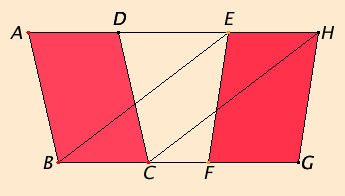# Proposition 36

Parallelograms which are on equal bases and in the same parallels equal one another.

Let ABCD and EFGH be parallelograms which are on the equal bases BC and FG and in the same parallels AH and BG.I say that the parallelogram ABCD equals EFGH.

I.Post.1

Join BE and CH.

Since BC equals FG and FG equals EH, therefore BC equals EH.

I.33

But they are also parallel, and EB and HC join them. But straight lines joining equal and parallel straight lines in the same directions are equal and parallel, therefore EBCH is a parallelogram.

I.35

And it equals ABCD, for it has the same base BC with it and is in the same parallels BC and AH with it.

C.N.1

For the same reason also EFGH equals the same EBCH, so that the parallelogram ABCD also equals EFGH.

Therefore parallelograms which are on equal bases and in the same parallels equal one another.

Q.E.D.

## Guide

This proposition is a generalization of the previous proposition I.35, and its proof depends directly on it. Euclid could have bundled the two propositions into one. Then the special case of I.35 would have been proven first and then used to prove the general case of I.36. In an introductory book like Book I this separation makes it easier to follow the logic, but in later books special cases are often bundled into the general proposition.

#### Use of Proposition 36

This proposition is used in I.38, a few propositions in Books II and VI, and XI.29